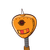# If the common zero of 3x²-px+2 and 4x²- qx- 10 is 2 , than find the value of 2p -3q.​

If the common zero of 3x²-px+2 and 4x²- qx- 10 is 2 , than find the value of 2p -3q.​

### 1 thought on “If the common zero of 3x²-px+2 and 4x²- qx- 10 is 2 , than find the value of 2p -3q.​”

1.5

Step-by-step explanation:

when x = 2 both the equations will be equated to zero.

3x²-px+2

=> 3(2)²-p(2)+2 = 0

=> 12 – 2p + 2 = 0

=> 14 – 2p = 0

=> 14 = 2p

=> p = 7

4x²-qx-10

=> 4(2)²-q(2)-10 = 0

=> 16-2q-10 = 0

=> 6-2q = 0

=> 6=2q

=> q = 3

2p – 3q => 2(7)-3(3) = 14-9 = 5